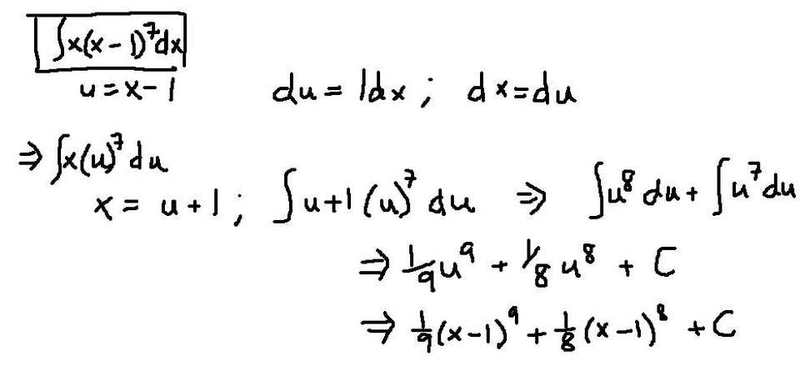# Anti-deriv (x(x-4)^7)

## Homework Statement## The Attempt at a Solution

Sorry, i didn't know how to put this on the forum so i did it on paint and uploaded it to tinypic.

Here is the image of the "attempted" solution.lanedance
Homework Helper
Best check is to differentiate

Last edited:
Oh, ok, but how do i differentiate the c and what do i do to bring back the x when i differentiate? (since i changed the x to u+1)

lanedance
Homework Helper
What's the derivative of a constant?

HallsofIvy
Homework Helper
Oh, ok, but how do i differentiate the c and what do i do to bring back the x when i differentiate? (since i changed the x to u+1)
The x is already "back": differentiate $(1/9)(x- 1)^9+ (1/8)(x- 1)^8+ C$.

Mentallic
Homework Helper
I'll be frank here, if you don't know what to do with the c then you probably don't have a clue why you put it there in the first place. You should go back and read up on the fundamentals of integration.

The x is already "back": differentiate $(1/9)(x- 1)^9+ (1/8)(x- 1)^8+ C$.
(x-1)8+(x-1)7 => (x-1)7x (im guessing the c is a constant?)

I'll be frank here, if you don't know what to do with the c then you probably don't have a clue why you put it there in the first place. You should go back and read up on the fundamentals of integration.

we never learned the fundamentals of integration :)
We are going to learn them today, this is just a problem she gave us and said to try to solve it on our own. (like a heads-up kind of thing)
I just looked up an example and tried to copy it and i really have no idea what c is. (so i guessed :D)

Mentallic
Homework Helper
Oh ok, in that case, yes the c is just a constant so whenever you take the derivative of a constant it is always 0.

Ok so are you satisfied? Did you get from (x-1)8+(x-1)7 => x(x-1)7 by some process or did you just assume it should be equal?

i actually assumed it should be equal :)
I'm really bad at simplifying, but i thought it should equal that.

Mentallic
Homework Helper
Well since you have two factors, (x-1)8 and (x-1)7 and you need to get to 1 factor, then you should factorize! Letting (x-1)7=u will make things a lot more easy to spot.

oh....

so then it would be u[x-1+1]?
then the -1 and 1 cancel out, and you get u[x]

Mentallic
Homework Helper
Yep :tongue:

great!! Thank you so muchHallsofIvy